# Texas Go Math Grade 7 Lesson 9.4 Answer Key Area of Composite Figures

Refer to our Texas Go Math Grade 7 Answer Key Pdf to score good marks in the exams. Test yourself by practicing the problems from Texas Go Math Grade 7 Lesson 9.4 Answer Key Area of Composite Figures.

## Texas Go Math Grade 7 Lesson 9.4 Answer Key Area of Composite Figures

Essential Question
How do you find the area of composite figures?

Exploring Areas of Composite Figures
Aaron was plotting the shape of his garden on grid paper. While it was an irregular shape, it was perfect for his yard. Each square on the grid represents 1 square meter.

A. Describe one way you can find the area of this garden.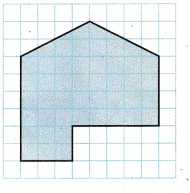B. The area of the garden is ___ square meters.

C. Compare your results with other students. What other methods were used to find the area?

D. How does the area you found compare with the area found using different methods?

Reflect

Question 1.
Use dotted lines to show two different ways Aaron’s garden could be divided up into simple geometric figures.Seperate figure into a triangle and two rectangles.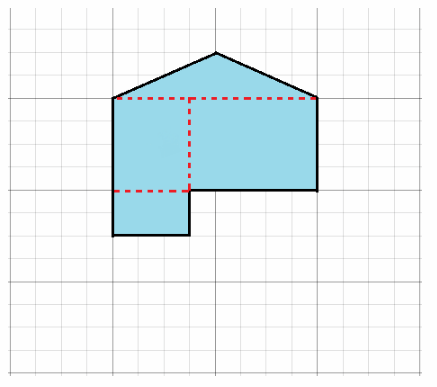Separate figure into a triangle and three rectangles.

One way is to separate figure into a triangle and two rectangles, and other way is to separate figure into a triangle and three rectangles

Finding the Area of a Composite Figure

A composite figure is made up of simple geometric shapes. To find the area of a composite figure or other irregular-shaped figure, divide it into simple, nonoverlapping figures. Find the area of each simpler figure, and then add the areas together to find the total area of the composite figure.
Use the chart below to review some common area formulas.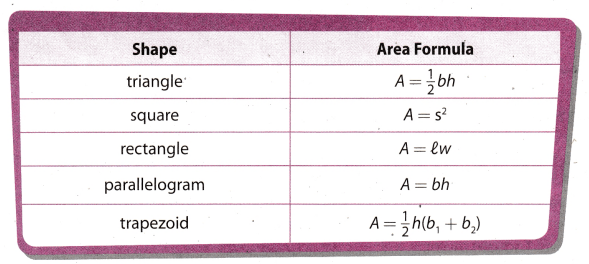Find the area of the figure.
Step 1
Separate the figure into smaller, familiar figures: a parallelogram and a trapezoid.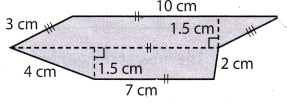Step 2
Find the area of each shape.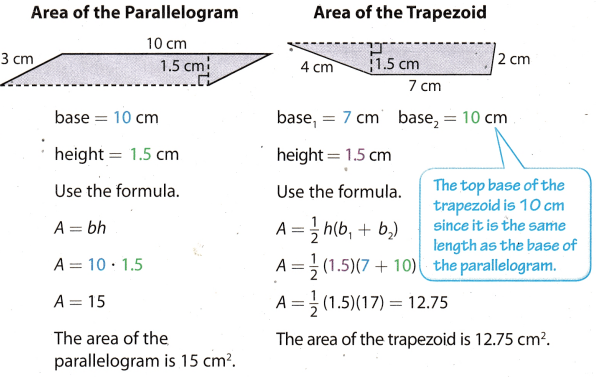Step 3
Add the areas to find the total area.
A = 15 + 12.75 = 27.75 cm2
The area of the figure is 27.75 cm2.

Find the area of each figure. Use 3.14 for π.

Question 2.Separate the figure into 2 triangles and one rectangle.
Area of the first triangle
base = 2 ft
height = 3 ft
Use the formula for the area of the triangle.
A1 = $$\frac{1}{2} \cdot b \cdot h$$
A1 = $$\frac{1}{2} \cdot 2 \cdot 3$$
A1 = $$\frac{6}{2}$$
A1 = 3
The area of the first triangle is 3 ft2.
Area of the second triangle
base = 3 ft
height = 3 ft
Use the forumula for the area of the triangle.
A2 = $$\frac{1}{2} \cdot b \cdot h$$
A2 = $$\frac{1}{2} \cdot 3 \cdot 3$$
A1 = $$\frac{9}{2}$$
A1 = 4.5
The area of the second triangle is 4.5 ft2.

Area of the rectangle
length = 8 ft
width = 4 ft
Use the forum ula for the area of the rectangle.
À3 = l ∙ w
A3 = 8 ∙ 4
A3 = 24
The area of the rectangle is 24 ft2.
Add the areas to find the total area.
A = A1 + A2 + A3 = 3 + 4.5 + 24 = 31.5
The area of the figure is 31.5 ft2.

Question 3.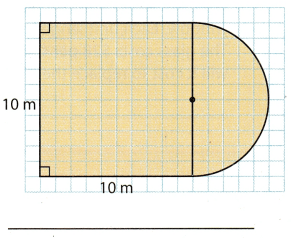Separate the figure into a square and a semicircle.

Area of the square
side = 10 m
Use the formula for the area of the square.
A1 = s2
A1 = 102
A1 = 100
The area of the sqaure is 100 m2.
Area of the circle
diameter = 10 m
Use the formula for the area of the circle when given diameter
Ac = $$\pi\left(\frac{d}{2}\right)^{2}$$ Subsitute 10 ford and 3.14 for π.
Ac = $$3.14\left(\frac{10}{2}\right)^{2}$$
Ac = 3.14 ∙ 52
Ac = 3.14 ∙ 25
Ac = 78.5
Area of the semicircle is half the area of the circle.
A2 = $$\frac{A_{c}}{2}$$ = $$\frac{78.5}{2}$$ = 39.25
The area of the semicircle is 39.25 m2.
Add the areas to find the total area.
A = A1 + A2 = 100 + 39.25 = 139.25
The area of the figure is 139.25 m2

Using Area to Solve Problems

Example 2
A banquet room is being carpeted. A floor plan of the room is shown at right. Each unit represents 1 yard. The carpet costs $23.50 per square yard. How much will it cost to carpet the room?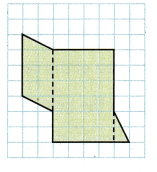Step 1 Separate the composite figure into simpler shapes as shown by the dashed lines: a parallelogram, a rectangle, and a triangle. Step 2 Find the area of the simpler figures. Count units to find the dimensions.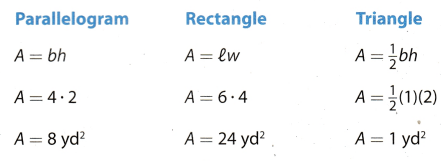Step 3 Find the area of the composite figure. A = 8 + 24 + 1 = 33 square yards Step 4 Calculate the cost to carpet the room. Area ∙ Cost per yard = Total cost 33 ∙$23.50 = $775.50 The cost to carpet the banquet room is$775.50.

Question 4.
A window is being replaced with tinted glass. The plan at the right shows the design of the window. Each unit length represents 1 foot. The glass costs $28 per square foot. How much will it cost to replace the glass? Use 3.14 for π.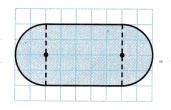Answer: Seperate the figure into a rectangle and two semicircles. Since each unit length represents 1 foot, we see from the picture that the diameter of the semicircles is 4 ft, and length and width of the rectangle are 5 ft and 4 ft. We have two semicircles with same diameter, which make up the whole circle. Hence, find the area of the circle. Area of the circle diameter = 4 Use the formula for the area of the circle when given diameter. A1 = $$\pi\left(\frac{d}{2}\right)^{2}$$ Substitute 4 for d and 3.14 for π. A1 = $$3.14\left(\frac{4}{2}\right)^{2}$$ A1 = 3.14 ∙ 22 A1 = 3.14 ∙ 4 A1 = 12.56 Area of the circle is 12.56 ft2. Area of the rectangle length = 5 ft width = 4 ft Use the formula for the area of the rectangle. A2 = l ∙ w A2 = 5 ∙ 4 A2 = 20 The area of the rectangle is 20 ft2. Add the areas to find the total area. A = A1 + A2 = 12.56 + 20 = 32.56 The area of the figure is 32.56 ft2.. To find how will it cost to replace the glass multiply the area of the figure by the cost of the glass per square foot. The total cost = 32.56 ∙ 28 = 911.68$911.68 will cost the replacement of the glass.

Question 1.
A tile installer plots an irregular shape on grid paper. Each square on the grid represents 1 square centimeter. What is the area of the irregular shape? (Explore Activity, Example 2)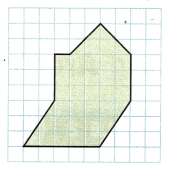Step 1
Separate the figure into a triangle, a ____ and a parallelogram.
Step 2
Find the area of each figure.
triangle: ____ cm2; rectangle: ___ cm2; parallelogram: ___ cm2
The area of the irregular shape is ____ cm2.
A rectangle
Area of the triangle
base = 4 cm
height = 2 cm
Use the formula for the area of the triangle.
A1 = $$\frac{1}{2} b \cdot h$$
A1 = $$\frac{1}{2} 4 \cdot 2$$
A1 = $$\frac{8}{2}$$
A1 = 4
The area of the triangle is 4 cm2.
Area of the rectangle
length = 5 cm
width = 3 cm
Use the formula for the area of the rectangle.
A2 = l ∙ w
A2 = 5 ∙ 3
A2 = 15
The area of the rectangle is 15 cm2
Area of the parallelogram
length = 5 cm
width = 3 cm
Use the formula for the area of the parallelogram.
A3 = l ∙ w
A3 = 5 ∙ 3
A3 = 15
The area of the parallelogram is 15 cm2.

Add the areas to find the total area.
A = A1 + A2 + A3 = 4 + 15 + 15 = 34
The area of the irregular shape is 34 cm2.

Question 2.
Show two different ways to divide the composite figure. Find the area both ways. Show your work below. (Example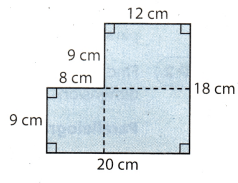First way
Separate the figure into two rectangles.
Area of the first rectangle
length = 20 cm
width = 9 cm
Use the formula for the area of the rectangle.
A1 = l ∙ w
A1 = 20 ∙ 9
A1 = 180
The area of the first rectangle is 180 cm2.
Area of the second rectangle
length = 12 cm
width = 9 cm
Use the formula for the area of the rectangle.
A2 = l ∙ w
A2 = 20 ∙ 9
A2 = 180
The area of the second rectangle is 108 cm2.
Add the areas to find the total area.
A = A1 + A2 = 180 + 108
The area of the figure is 288 cm2.

Second way
Separate the figure into three rectangles.
Area of the first rectangle
length = 9 cm
width = 8 cm
Use the formula for the area of the rectangle.
A1 = l ∙ w
A1 = 9 ∙ 8
A1 = 78

The area of the first rectangle is 72 cm2.
Area of the second rectangle
length = 12 cm
width = 9 cm
Use the formula for the area of the rectangle.
A2 = l ∙ w
A2 = 12 ∙ 9
A2 = 108
The area of the second rectangle is 108 cm2.

Area of the third rectangle
length = 12 cm
width = 9 cm
Use the formula for the area of the rectangle.
A2 = l ∙ w
A2 = 12 ∙ 9
A2 = 108
The area of the third rectangle is 108 cm2.

Add the areas to find the total area.
A = A1 + A2 + A3 = 72 + 108 + 108 = 288
The area of the figure is 288 cm2.

Question 3.
Sal is tiling his entryway. The floor plan is drawn on a unit grid. Each unit length represents 1 foot. Tile costs $2.25 per square foot. How much will Sal pay to tile his entryway? (Example 2)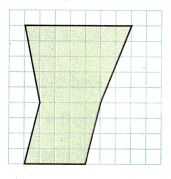Answer: Separate the figure into a parallelogram and a trapezoid. Area of the parallelogram length = 4 ft width = 4 ft Use the formula for the area of the parallelogram. A1 = l ∙ w A1 = 4 ∙ 4 A1 = 16 The area of the parallelogram is 16 ft2. Area of the trapezoid b1 = 7 ft b2 = 4 ft h = 5 ft Use the formula for the area of the trapezoid.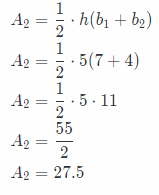The area of the trapezoid is 27.5 ft2. Add the areas to find the total area. A = A1 + A2 = 16 + 27.5 = 43.5 The area of the figure is 43.5 ft2. The total area of the floor is 43.3 ft2. Total cost of tilling = 43.5 ∙ 2.25 = 97.87 Sal will pay$97.87 to tile his entryway.

Essential Question Check-In

Question 4.
What is the first step in finding the area of a composite figure?
First step is to separate the composite figure into smaller familiar figures.

Question 5.
A banner is made of a square and a semicircle. The square has side lengths of 26 inches. One side of the square is also the diameter of the semicircle. What is the total area of the banner? Use 3.14 for π.
Area of the square
side = 26 in.
Use the formula for the area of the square.
A1 = s2
A1 = 262
A1 = 676
The area of the sqaure is 676 in.2.
Area of the circle
diameter = 26 in.
Use the formula for the area of the circle when given diameter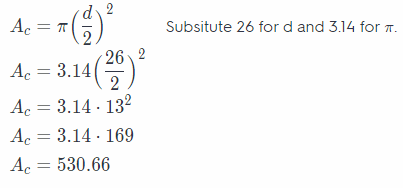Area of the semicircle is half the area of the circle.
A2 = $$\frac{A_{c}}{2}$$ = $$\frac{530.66}{2}$$ = 265.33
The area of the semicircle is 265.33 in.2.

Add the areas to find the total area.
A = A1 + A2 = 676 + 265.33 = 941.33
The area of the banner is 941.33 in.2

Question 6.
Multistep Erin wants to carpet the floor of her closet. A floor plan of the closet is shown.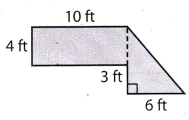Separate the floor into a rectangle and a triangle.
Area of the triangle
base = 6 ft
height = 7 ft
Use the formula for the area of the triangle.
A1 = $$\frac{1}{2} b \cdot h$$
A1 = $$\frac{1}{2} 6 \cdot 7$$
A1 = $$\frac{42}{2}$$
A1 = 21
The area of the triangle is 21 ft2.
Area of the rectangle
length = 10 ft
width = 4 ft
Use the formul.a for the area of the rectangle
A3 = l ∙ w
A3 = 10 ∙ 4
A3 =40
The area of the rectangle is 40 ft2.

Add the areas to find the total area.
A = A1 + A2 = 21 + 40 = 61
The area of the floor is 61 ft2.

a. How much carpet does Erin need?
The area of the floor is 61 ft2.

b. The carpet Erin has chosen costs $2.50 per square foot. How much will it cost her to carpet the floor? Answer: To find the total cost of the carpet multiply total area of the carpet by the cost of carpet per square foot. Total cost of the carpet = 61 ∙ 2.5 = 152.5 Total cost of the carpet =$152.5

Question 7.
Multiple Representations Hexagon ABCDEF has vertices A(-2, 4), B(0, 4), C(2, 1), D(5, 1), E(5, -2), and F(-2, -2). Sketch the figure on a coordinate plane. What is the area of the hexagon?The area of hexagon consists of rectangle and trapezoid

Question 8.
A field is shaped like the figure shown. What is the area of the field? Use 3.14 for π.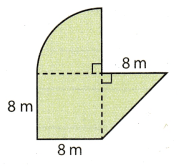Separate the field into a triangle, a square and a quarter of a circle.
Area of the triangle
base = 8 m
height = 8 m
Use the formula for the area of the triangle.
A1 = $$\frac{1}{2} b \cdot h$$
A1 = $$\frac{1}{2} 8 \cdot 8$$
A1 = $$\frac{64}{2}$$
A1 = 32
The area of the triangle is 32 m2.
Area of the square
side = 8 m
Use the formula for the area of the square.
A2 = s2
A2 = 82
A2 = 64
The area of the sqaure is 64 m2.

To find the area of the quarter of a circle, find the area of the circle and divide it by 4.
Area of the circle
Use the formula for the area of the circle when given radius.
Ac = π(r)2 Substitute 10 for r and 3.14 for π.
Ac = 3.14(8)2
Ac = 3.14 ∙ 64
Ac = 200.96
A3 = $$\frac{A_{c}}{4}$$ = $$\frac{200.96}{4}$$ = 50.24
The area of the quarter of the circle is 50.24 m2.
Add the areas to find the total area.
A = A1 + A2 + A3 = 32 + 64 + 50.24 = 146.24
The area of the field is 146.24 m2.

Question 9.
A bookmark is shaped like a rectangle with a semicircle attached at both ends. The rectangle is 12 cm long and 4 cm wide. The diameter of each semicircle is the width of the rectangle. What is the area of the bookmark? Use 3.14 for π.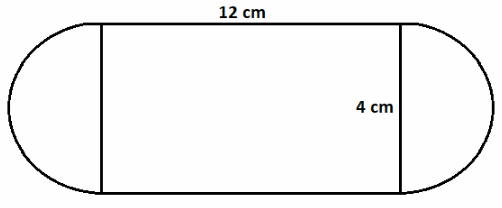Separate the figure into a rectangle and two semicircles.
Two semicircles with the same diameter make a circle with the same diameter
Area of the circle
diameter = 4 cm
Use the formula for the area of the circle when given diameter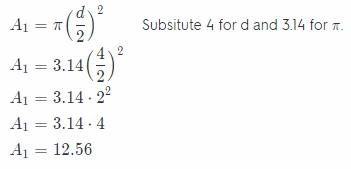Area of the circle is 12.56 cm2.
Area of the rectangle
length = 12 cm
width = 4 cm
Use the formula for the area of the rectangle.
A2 = l ∙ w
A2 = 12 ∙ 4
A2 = 48
The area of the rectangle is 48 cm2.
Add the areas to find the total area.
A = À1 + À2 = 12.56 + 48 = 60.56
The area of the bookmark is 60.56 cm2

Question 10.
Multistep Alex is making 12 pennants for the school fair. The pattern he is using to make the pennants is shown in the figure. The fabric for the pennants costs $1.25 per square foot. How much will it cost Alex to make 12 pennants?Answer: Separate the figure into a triangles and one rectangle. Area of the triangle base = 1 ft height = 1 ft Use the formula for the area of the triangle.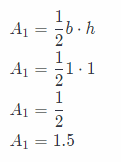The area of the triangle is 1.5 ft2. Area of the rectangle length = 3 ft width = 1 ft Use the formula for the area of the rectangle. A2 = l ∙ w A2 = 3 ∙ 1 A2 = 3 The area of the rectangLe is 3 ft2. Add the area to find the total area. A = A1 + A2 = 1.5 + 3 = 4.5 The area of the pennants is 4.5 ft2. The cost of one pennants = 4 ∙ 1 .25 = 5 The cost of one pennants is 85. Alex is making 12 of them so the total cost of 12 is The total cost = total cost of one pennants∙12 = 5 ∙ 12 = 60 Alex will pay$60 for 12 pennants.

Question 11.
Reasoning A composite figure is formed by combining a square and a triangle. Its total area is 32.5 ft2. The area of the triangle is 7.5 ft2. What is the length of each side of the square? Explain.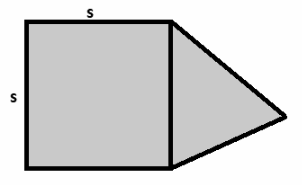A = the area of the composite figure
A1 = the area of the triangle
A2 = the area of the square
A1 = 7.5ft
A = 32.5 ft
Composite figure consists a triangle and a square.
A = A1 + A2 Substitute 7.5 for A_1 and 32.5 for A.
32.5 = 7.5 + A2 Subtract 7.5 from both sides.
32.5 – 7.5 = A2
A2 = 25
The area of the square is 25 ft2.
A2 = s2. Substititute 25 for A_2
25 = s2 Root both sides.
$$\sqrt{25}$$ = $$\sqrt{s^{2}}$$
5 = s
s = 5
The Length of side of the square is 5 ft

Texas Go Math Grade 7 Lesson 9.4 H.O.T. Focus On Higher Order Thinking Answer Key

Question 12.
Represent Real-World Problems Christina plotted the shape of her garden on graph paper. She estimates that she will get about 15 carrots from each square unit. She plans to use the entire garden for carrots. About how many carrots can she expect to grow? Explain.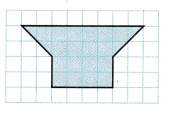Separate the figure into a rectangle and a trapezoid.
Area of the parallelogram
length = 4
width = 2
Use the formula for the area of the rectangle.
A1 = l ∙ w
A1 = 4 ∙ 2
A1 = 8
The area of the parallelogram is 8 square units.
Area of the trapezoid
b1 = 8
b2 = 6
h = 2
Use the formula for the area of the trapezoid.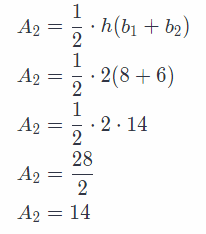Add the areas to find the total area.
A = A1 + A2 = 8 + 14 = 22
The area of the garden is 22 square units.
Christina estimates that she’ll get 15 carrots from each square unit hence, total number of carrots she can expect
is 22 ∙ 15 = 330.

Total number of carrots she can expect is 330.

Question 13.
Analyze Relationships The figure shown is made up of a triangle and a square. The perimeter of the figure is 56 inches. What is the area of the figure? Explain.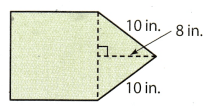The perimeter of the figure consists 3 sides of a square and 2 sides of a triangle.
Hence
P = 3 s1 – 2 s2
where s1 represents side of a square, and s2 represents side of a triangle.
P = 56 in.
s2 = 10 in.
P = 3 ∙ s1 + 2 ∙ s2 Substitute 56 for P, and 10 for s_2.
56 = 3s1 + 2 ∙ 10
56 = 3s1 + 20 Subtract 20 from both sides.
56 – 20 = 3s1
36 = 3s1 Divide both sides by 3.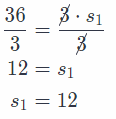The side of the square is 12 in.
The side of the square is also base of the triangle.

Area of the triangle
base = 12 in.
height = 8 in.
Use the formula for the area of the triangle.The area of the triangle is 48 in.2.
Area of the square
side = 12 in.
Use the formula for the area of the square.
A2 = s2
A2 = 122
A2 = 144
The area of the square is 144 in.2.
Add the areas to find the total area.
A = A1 + A2 = 48 + 144 = 192
The area of the figure is 192 in.2.

Question 14.
Critical Thinking The pattern for a scarf is 28 in. shown at right. What is the area of the scarf? Use 3.14 for π.The pattern for a scarf we get when we subtract two semicircles with the diameter 15 in. from the rectangle with 28 in. length and 15 in.width.
Hence, the area of the scarf is
A = A1 – A2
A1 = the area of the rectangle
A2 = area of the two semicircles with same diameter, which make a circle with the same diameter.
Area of the rectangle
Length = 28 in.
width = 15 in.
Use the formula for the area of the rectangle.
A1 = l ∙ w
A1 = 28 ∙ 15
A1 = 420
The area of the rectangle is 420 in 2

Area of the circle
diameter = 15 in.
Use the formula for the area of the circle when given diameter.Area of the circle is 176.62 in.2.
A = A1 – A2 = 420 – 176.62 = 243.38
The area of the scarf is 243.38 in.2.

Question 15.
Persevere in Problem Solving The design for the palladium window shown includes a semicircular shape at the top. The bottom is formed by squares of equal size. A shade for the window will extend 4 inches beyond the perimeter of the window, shown by the dashed line around the window. Each square in the window has an area of 100 in2.

a. What is the area of the window? Use 3.14 for π.
The length of the side of small, squares is 10 inches since their area is loo square inches. Since there are four small
squares for the square part of the palladium window, the length will be 40 inches. Determine the area of the
square part of the window.
A = s2 Write the formula for the area of square
A = 402 Substitute the value
A = 1, 600 Evaluate the exponent
The diameter of the semicircle is 40 inches (Length of the square of the window). The radius is half of the diameter,
therefore, the radius is 20 inches. Determine the area of the semicircle.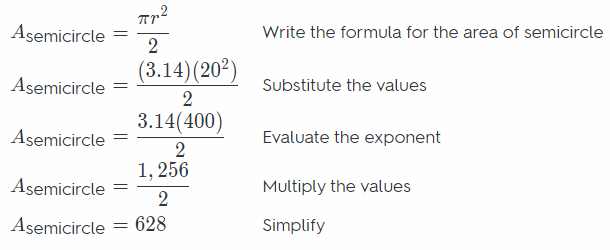Determine the area of the window.
Awindow = Asquare + Asemicircle
Awindow = 1, 600 in2 + 628 in2
Awindow = 2, 228 in2

From the length of the window which is 40 inches, adding the shadow will give the dimensions of the square part
of the window as 48 inches by 44 inches. Determine the area of the rectangle part of the window
A = lw Write the formula for the area of rectangle
A = 48(44) Substitute the value
A = 2, 112 Multiply the values
The diameter of the semicircle with the shadow will be 48 inches. The radius is half of the diameter, therefore, the
radius is 24 inches. Determine the area of the semicircle.Determine the area of the shade.